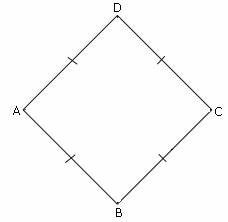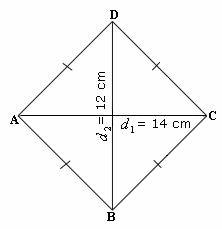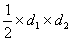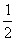## Definition Of Diamond

Diamond is a parallelogram with four equal sides.

### More About Diamond

Another name for diamond is rhombus, so all the properties of rhombus hold true for diamond.

### Example of DiamondIn the above figure, the four sides namely AB, BC, CD, DA are parallel and equal. So, ABCD is a diamond.

### Solved Example on Diamond

#### Ques: Find the area of a diamond with diagonals 14 cm and 12 cm.##### Choices:

A. 84 cm2
B. 26 cm2
C. 13 cm2
D. 64 cm2
Step 1: Area of diamond =[Formula.]
Step 2: =× 14 × 12 [Substitute the values of d1 and d2.]